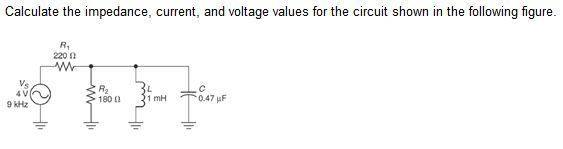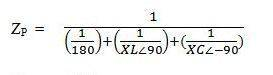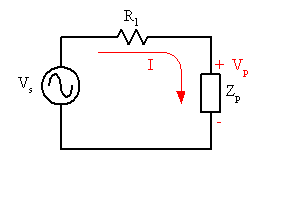# Impedance for series-parallel RLC circuit problem

• Engineering
damien2
This is my first time posting so forgive me if I screw it up. I have attempted to attach the file, I hope I have done so correctly.
I am trying to solve a series-parallel RLC circuit problem. I have a 220Ω resistor in series with 180Ω resistor, a 1mH inductor and a .47μF capacitor all in parallel. source voltage is equal to 4V at 9kHz.

## Homework Equationsthis is what I have done so far.
XC=1/2π(9000)(.00000047)=37.6
XL= 2π(9000)(.001)
ZP¬=1/((1/180)+(1/37.6)+(1/56.6))=20.07

Mentor
This is my first time posting so forgive me if I screw it up. I have attempted to attach the file, I hope I have done so correctly.
I am trying to solve a series-parallel RLC circuit problem. I have a 220Ω resistor in series with 180Ω resistor, a 1mH inductor and a .47μF capacitor all in parallel. source voltage is equal to 4V at 9kHz.

## Homework Equations

View attachment 50607

this is what I have done so far.
XC=1/2π(9000)(.00000047)=37.6
XL= 2π(9000)(.001)
ZP¬=1/((1/180)+(1/37.6)+(1/56.6))=20.07

Hi damien2, Welcome to Physics Forums.

Keep in mind that impedances are complex quantities (real + imaginary components). For the reactive components (capacitor, inductor), the reactances you've calculated above (XC, XL) are the magnitudes of their impedances without the "angle" information.

Include the imaginary constant j where appropriate and then carry out the complex arithmetic.

damien2
gneill, I would like to thank you for taking the time to post a response to my thread. Unfortunately I would need more than that to get any further in this problem. There are many different formulas and I am just lost.
I have been trying to figure this problem out since Thursday. I have spent over 14 hours looking for an example to show me how to solve a series-parallel RLC circuit. I know that I am missing a lot of pieces here, and I am not sure what the angle is anymore.
I am taking this course online so no professor and no teaching. You Just read the book and figure it out yourself. Well there is no clear example or explanation in the book for how and what to do and no Instructor to ask for help.

damien 2

Mentor
The intrinsic impedances of the components are:

ZR = R
ZL = jωL
ZC = 1/(jωC)

where ##j = \sqrt{-1}##

These impedances can be manipulated just like resistance values in all the usual analysis formulas, the difference being that you follow the rules of complex arithmetic.

So, if you consider just the components R2, L, and C, can you determine the net impedance for their parallel connection?

damien2
gneill,
I do appreciate you trying to help but I already solved for Zp isn't that my impedance of the parallel combination? I need to determine the formula to get the phase angle, figure out how to convert Zp into rectangular form. Once I have Zp in rectangular form I can solve for Zt(=Zp+R1). Convert that into polar form; use that to find the I(=Vs/Zt). After 14 hours on this problem I would bet on my chances to solve a simple division problem. I am beyond frustrated so forgive me for not understanding or not seeming appreciative, I am toast.

Mentor
gneill,
I do appreciate you trying to help but I already solved for Zp isn't that my impedance of the parallel combination? I need to determine the formula to get the phase angle, figure out how to convert Zp into rectangular form. Once I have Zp in rectangular form I can solve for Zt(=Zp+R1). Convert that into polar form; use that to find the I(=Vs/Zt). After 14 hours on this problem I would bet on my chances to solve a simple division problem. I am beyond frustrated so forgive me for not understanding or not seeming appreciative, I am toast.

Use the complex impedances of the components to determine Zp. The result will be a complex value from which you can extract both the magnitude and angle (convert the rectangular form to polar form).

You can't combine reactances in the way you did to find the parallel impedance; it doesn't take into account relative 'angles' of the individual impedances. This is akin to why you can't just add the magnitudes of complex numbers to obtain the overall magnitude of the sum. You have to carry through the complex arithmetic and then find the magnitude of the result.

damien2that is where my confusion begins, I know that Xl is at ∠90 and Xc∠-90 but I need help on properly completing the formulas. Is there a website a breakdown of the process with the formulas that you could direct me to. I need to see how this problem is completed from start to finish.

Mentor
View attachment 50612
that is where my confusion begins, I know that Xl is at ∠90 and Xc∠-90 but I need help on properly completing the formulas.
That's why you need to use the complex values for the component impedances. The complex arithmetic automatically takes care of the angles. Just plug in the numbers. In your attachment where you've got XL∠90 insert jXL. In place of XC∠-90 insert -jXC. Simplify to a single complex value.
Is there a website a breakdown of the process with the formulas that you could direct me to. I need to see how this problem is completed from start to finish.

Do a web search on "adding impedances" and you should turn up a number of references.

damien2
thanks for the help gneill, unfortunately I don't believe you realize that this is my first AC circuit class and I have never taken a math class above algebra. You keep saying to solve for this or that but the problem is I don't know the formula or procedure to do that. I just trying to dissect the problem I have so I can see the process. You are saying to solve for intrinsic impedence, That method has not been used in the book yet. Formulas and steps are what I need. I need the for dummies version.

Mentor
I spelled out the intrinsic impedances for the components in post #4. These are the things that you plug in in place of 'resistance' in all the usual circuit analysis formulas (like Ohm's law, etc.).

Have you not covered complex arithmetic? If not, there are web-based tutorials if you do a search for "complex arithmetic tutorial". There are also online complex arithmetic calculators (to take the drudgery out of doing it by hand).

Once you have the impedance for the parallel components you'll be left with a voltage divider configuration:From this you can find Vp, the voltage across the parallel components. With that and their individual impedances you can calculate their individual currents using Ohm's law.